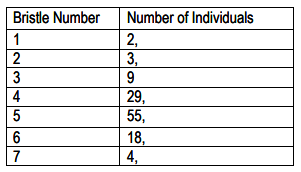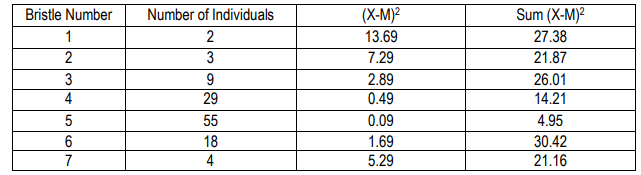Clutch Prep is now a part of Pearson
Ch.20 Quantitative GeneticsWorksheetSee all chapters

# Mathematical Measurements

See all sections
Sections
Mathematical Measurements
Traits and Variance
Analyzing Trait Variance
Heritability
QTL Mapping

Concept #1: Mathematical Measurements

###### Expert Q&A

Ask unlimited questions and get expert help right away.

Practice: The table shows a distribution of bristle numbers in a Drosophila population. What is the mean bristle number?Practice: The table shows a distribution of bristle numbers in a Drosophila population. What is the variance?Practice: Using the variance calculated in problem #2, what is the standard deviation?

###### Expert Q&A

Ask unlimited questions and get expert help right away.